knn 机器学习_机器学习knn - CSDN
• # _*_ coding _*_ import numpy as np import math import operator def get_data(dataset): x = dataset[:,:-1].astype(np.float) y = dataset[:,-1] return x,y # def cal_dis(a,b): # x1,y1 = ...
# _*_ coding _*_
import numpy as np
import math
import operator

def get_data(dataset):
x = dataset[:,:-1].astype(np.float)
y = dataset[:,-1]
return x,y
# def cal_dis(a,b):
#     x1,y1 = a[:]
#     x2,y2 = b[:]
#     dist = math.sqrt(math.pow(2,x2)-math.pow(2,x1))

def knnclassifer(dataset,predict,k=3):
x,y = get_data(dataset)
dic = {}
distince = np.sum((predict-x)**2,axis=1)**0.5
sorted_dict = np.argsort(distince)#[2 1 0 3 4]
countLabel = {}
for i in range(k):
label = y[sorted_dict[i]]
#  print(i,sorted_dict[i],label)
countLabel[label] = countLabel.get(label,0)+1
new_dic = sorted(countLabel,key=operator.itemgetter(0),reverse=True)
return new_dic

if __name__ == '__main__':

predict = [2,2]
label  = knnclassifer(dataset,predict,3)
print(label)


展开全文机器学习
• 最近在学习机器学习算法，感觉有本书写得很不错——《机器学习实战》，如果有一点基础去看这本书，然后在结合书中实例进行实践，还是很有收获的。 之后，可能会不定时的更新此书的相关内容，主要内容参考此书，...
最近在学习机器学习算法，感觉有本书写得很不错——《机器学习实战》，如果有一点基础去看这本书，然后在结合书中实例进行实践，还是很有收获的。

之后，可能会不定时的更新此书的相关内容，主要内容参考此书，夹杂一些我自己实践中的经验。

KNN算法

本书介绍的第一个机器学习算法是kNN算法，这个算法比较基础，简单易懂。

实现的主要步骤：
(1)收集数据。
(2)准备数据：使用python解析文本文件
(3)分析数据：主要是可视化数据
(4)训练算法：kNN不用训练
(5)测试算法：计算错误率，或者准确率
(6)使用算法：
A. 输入训练样本向量或者特征向量；
B. 计算待分类样本（或特征向量）到这些训练样本的距离，一般是欧式距离
C. 选取最小的k个距离，统计对应的类别，哪个类别多，待分类的样本就属于哪一类

kNN的简单实现

注：代码主要参考自书本，有一些改动。

kNN的简单实现

#-*- coding:utf-8 -*-
import numpy as np
import matplotlib.pyplot as plt
import os
import cv2 as cv

# kNN算法的流程
# 收集、整理数据->计算数据到分类点的距离
# 选取k个距离最近的数据，看这几个数据属于哪一类，
# 所属类别多的，待分类的点就属于此类
# kNN算法不需要训练！！

def createDataSet():
# 构造简单的数据集
group = np.array([[1.0, 1.1],[1.0,1.0],[0,0.2],[0,0.1]])
# 这里对数据集进行修改，感觉书上的有点不合理 [0,0]->[0,0.2]
labels = ['A','A','B','B']
return group, labels

数据的可视化

def visualData(group,labels):
# 数据可视化
x1 = group[:2,0]
y1 = group[:2,1]
x2 = group[2:,0]
y2 = group[2:,1]
p1= plt.scatter(x1,y1)
p2= plt.scatter(x2,y2)
plt.legend([p1,p2],["A","B"])
plt.show()

def classify0(inX, dataSet, labels, k):
"""
:param inX: 待分类的向量 - [x0,y0]
:param dataSet: 数据集 - [[x1,y1],[x2,y2],...] - numpy类型
:param labels: 标签数据 - 'A'或者'B'
:param k: 设置的k值，选取前k个结果 - int
:return:分类的结果，'A'或者'B'
"""
d = inX-dataSet
sqMat = d**2
sqDis = sqMat.sum(axis=1)
distances = sqDis**0.5
sortedDis = distances.argsort()  # 将距离排序，并提取其对应的序号
assert len(sortedDis)>=k  # 检查k的设置是否有问题
classCount = {}
for i in range(k):
votelabel = labels[sortedDis[i]]
classCount[votelabel] = classCount.get(votelabel, 0)+1
keylist = list(classCount.keys())
s = 0
for l in keylist:
if classCount[l] > s:
s = classCount[l]
res = l
return res

运行结果：

可视化的结果：最终分类结果：kNN的手写数字识别

思路：将手写数字图像转为向量，然后计算测试图像向量和训练图像向量的距离，然后选k个所属类别最多的作为最终类别。

# 以下为手写数字识别的内容
def img2vector(filename):
# 将二进制图转换为向量，方便进行距离的计算
resVec = np.zeros((1, 1024))
fr = open(filename)
for i in range(32):
for j in range(32):
resVec[0, 32*i+j] = int(lineStr[j])
return resVec

def visualImg(filename):
# 可视化手写数字图像
resimg = np.zeros((32, 32))
fr = open(filename)
for i in range(32):
for j in range(32):
resimg[i][j] = int(lineStr[j])
cv.imshow("numberImg", resimg)
cv.waitKey(0)
return resimg

def handwritingClassTest():
hwLabels = []
trainingFileList = os.listdir('./digits/trainingDigits/')
m = len(trainingFileList)
trainingMat = np.zeros((m, 1024))
for i in range(m):
fileNameStr = trainingFileList[i]
fileStr = fileNameStr.split('.')
classNumStr = int(fileStr.split('_'))
hwLabels.append(classNumStr)
trainingMat[i,:] = img2vector('./digits/trainingDigits/%s' % fileNameStr)
testFileList = os.listdir('./digits/testDigits')
errorCount = 0.0
mTest = len(testFileList)
for i in range(mTest):
fileNameStr = testFileList[i]
fileStr = fileNameStr.split('.')
classNumStr = int(fileStr.split("_"))
vectorUnderTest = img2vector('./digits/testDigits/%s' % fileNameStr)
classifierResult = classify0(vectorUnderTest, trainingMat, hwLabels, 3)  # 计算测试集的向量和训练集的距离
print("the classifier came back with: %d, the real answer is %d"%(classifierResult, classNumStr))
if classifierResult!=classNumStr:
errorCount+=1.0
print("\nthe total number of errors is: %d"%errorCount)
print("\nthe total error rate is: %f"%(errorCount/float(mTest)))

手写数字图像的可视化：最终的分类结果：单看这个结果，好像比用CNN的结果还要好，https://blog.csdn.net/louishao/article/details/60867339
但是，kNN分类的这个数据集是比较小的，而且算法较为简单，比较复杂的情况分类的效果就会变得差一些，而且kNN无法表示图像/数据的特征。

完整代码

#-*- coding:utf-8 -*-
import numpy as np
import matplotlib.pyplot as plt
import os
import cv2 as cv

# kNN算法的流程
# 收集、整理数据->计算数据到分类点的距离
# 选取k个距离最近的数据，看这几个数据属于哪一类，
# 所属类别多的，待分类的点就属于此类
# kNN算法不需要训练！！

def createDataSet():
# 构造简单的数据集
group = np.array([[1.0, 1.1],[1.0,1.0],[0,0.2],[0,0.1]])
# 这里对数据集进行修改，感觉书上的有点不合理 [0,0]->[0,0.2]
labels = ['A','A','B','B']
return group, labels
def visualData(group,labels):
# 数据可视化
x1 = group[:2,0]
y1 = group[:2,1]
x2 = group[2:,0]
y2 = group[2:,1]
p1= plt.scatter(x1,y1)
p2= plt.scatter(x2,y2)
plt.legend([p1,p2],["A","B"])
plt.show()

def classify0(inX, dataSet, labels, k):
"""
:param inX: 待分类的向量 - [x0,y0]
:param dataSet: 数据集 - [[x1,y1],[x2,y2],...] - numpy类型
:param labels: 标签数据 - 'A'或者'B'
:param k: 设置的k值，选取前k个结果 - int
:return:分类的结果，'A'或者'B'
"""
d = inX-dataSet
sqMat = d**2
sqDis = sqMat.sum(axis=1)
distances = sqDis**0.5
sortedDis = distances.argsort()  # 将距离排序，并提取其对应的序号
assert len(sortedDis)>=k  # 检查k的设置是否有问题
classCount = {}
for i in range(k):
votelabel = labels[sortedDis[i]]
classCount[votelabel] = classCount.get(votelabel, 0)+1
keylist = list(classCount.keys())
s = 0
for l in keylist:
if classCount[l] > s:
s = classCount[l]
res = l
return res

# 以下为手写数字识别的内容
def img2vector(filename):
# 将二进制图转换为向量，方便进行距离的计算
resVec = np.zeros((1, 1024))
fr = open(filename)
for i in range(32):
for j in range(32):
resVec[0, 32*i+j] = int(lineStr[j])
return resVec

def visualImg(filename):
# 可视化手写数字图像
resimg = np.zeros((32, 32))
fr = open(filename)
for i in range(32):
for j in range(32):
resimg[i][j] = int(lineStr[j])
cv.imshow("numberImg", resimg)
cv.waitKey(0)
return resimg

def handwritingClassTest():
hwLabels = []
trainingFileList = os.listdir('./digits/trainingDigits/')
m = len(trainingFileList)
trainingMat = np.zeros((m, 1024))
for i in range(m):
fileNameStr = trainingFileList[i]
fileStr = fileNameStr.split('.')
classNumStr = int(fileStr.split('_'))
hwLabels.append(classNumStr)
trainingMat[i,:] = img2vector('./digits/trainingDigits/%s' % fileNameStr)
testFileList = os.listdir('./digits/testDigits')
errorCount = 0.0
mTest = len(testFileList)
for i in range(mTest):
fileNameStr = testFileList[i]
fileStr = fileNameStr.split('.')
classNumStr = int(fileStr.split("_"))
vectorUnderTest = img2vector('./digits/testDigits/%s' % fileNameStr)
classifierResult = classify0(vectorUnderTest, trainingMat, hwLabels, 3)  # 计算测试集的向量和训练集的距离
print("the classifier came back with: %d, the real answer is %d"%(classifierResult, classNumStr))
if classifierResult!=classNumStr:
errorCount+=1.0
print("\nthe total number of errors is: %d"%errorCount)
print("\nthe total error rate is: %f"%(errorCount/float(mTest)))

if __name__ == '__main__':
'''
group, labels = createDataSet()
visualData(group, labels)
inX = [0, 0]
classres = classify0(inX, group, labels, 3)
print(classres)
'''
'''
handWriteTrainDir = "./digits/trainingDigits/"
handWriteTestDir = "./digits/testDigits/"
trainlist = os.listdir(handWriteTestDir)
visualImg(handWriteTrainDir+trainlist)
vec = img2vector(handWriteTrainDir+trainlist)
'''
handwritingClassTest()
展开全文Python
• 简单概述：k-近邻算法采用测量不同特征值之间的距离方法进行分类。 k-近邻算法的一般流程 对未知类别属性的数据集中的每个点依次执行以下操作： (1)计算已知类别数据集中的点与当前点之间的距离；...
简单概述：k-近邻算法采用测量不同特征值之间的距离方法进行分类。

k-近邻算法的一般流程

对未知类别属性的数据集中的每个点依次执行以下操作：

(1)计算已知类别数据集中的点与当前点之间的距离；

(2)按照距离递增次序排序；

(3)选取与当前点距离最小的几个点；

(4)确定前k个点所在类别的出现频率；

(5)返回前k个点出现频率最高的类别作为当前点的预测分类。

如下图所示，有两类不同的训练样本数据，分别用蓝色的小正方形和红色的小三角形表示，而图正中间的那个绿色的圆所标示的数据则是待分类的数据。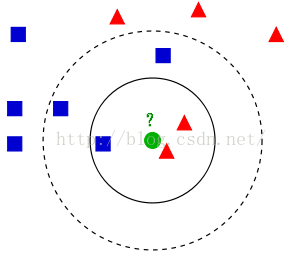衡量待分类样本的周围的k个邻居的类别，k个邻居中哪个类别较多，就把该类别判给待分类样本。（K近邻思想）

【注意】：当然K不同，待分类样本被判别的类别也可能不同。

优缺点

优点：精度高，对异常值不敏感，无数据输入假定（没有初始值）

缺点：计算复杂度高、空间复杂度高

适用数据范围：数值型和标称型（离散型数据，变量的结果只在有限目标集中取值）

工作原理是

存在一个样本数据集合，也称作训练样本集，并且样本集中每个数据都存在标签，即我们知道样本集中每一数据与所属分类的对应关系。输人没有标签的新数据后，将新数据的每个特征与样本集中数据对应的特征进行比较，然后算法提取样本集中特征最相似数据（最近邻）的分类标签。一般来说，我们只选择样本数据集中前k个最相似的数据，这就是k-近邻算法中k的出处,通常k是不大于20的整数。最后，选择k个最相似数据中出现次数最多的分类，作为新数据的分类。

距离计算方法

K-近邻算法的核心在于找到实例点的邻居。要找邻居就要度量相似性。估量不同样本之间的相似性，通常采用的方法就是计算样本间的“距离”，相似性度量方法有：欧式距离、余弦夹角、曼哈顿距离、切比雪夫距离等。

1.欧式距离：欧式距离来源于欧式空间中的两点之间的距离公式。

二维平面上的两点：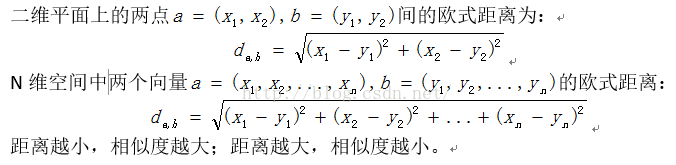2.曼哈顿距离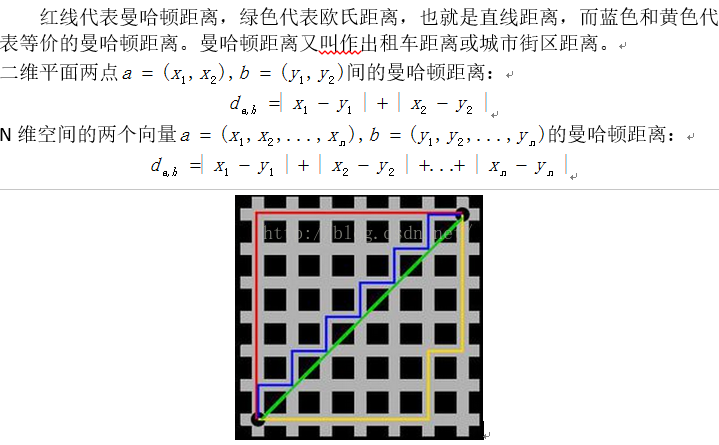3.切比雪夫距离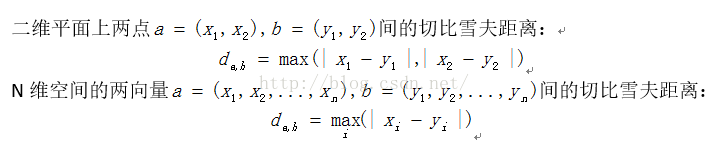4.余弦距离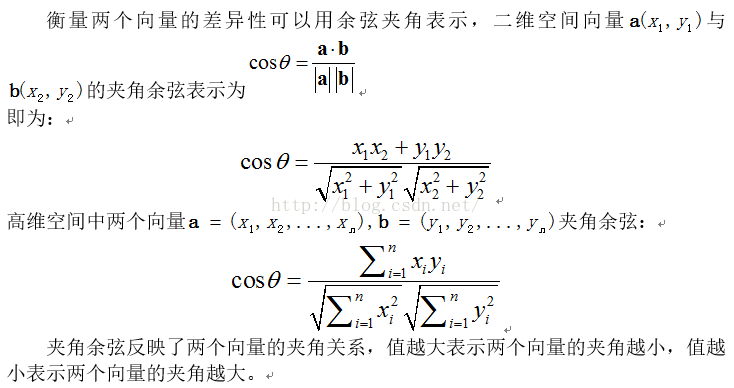K值选择

若k值较小，只有与输入实例较近（相似）的训练实例才会对预测结果起作用，预测结果会对近邻实例点非常敏感。如果近邻实例点恰巧是噪声，预测就会出错。容易发生过拟合。

若k较大，与输入实例较远的（不相似的）训练实例也会对预测起作用，容易使预测出错。k值的增大就意味着整体的模型变简单。

在应用中，k值一般取较小值。通常通过经验或交叉验证法来选取最优的k值。

分类决策规则

投票表决

少数服从多数，输入实例的k个近邻中哪个类的实例点最多，就分为该类。

加权投票法

根据距离的远近，对K个近邻的投票进行加权，距离越近则权重越大（比如权重为距离的倒数）。

归一化特征值

某一列的特征数值远远大于其他特征，这样在求距离的公式中就会占很大的比重，致使两点的距离很大程度上取决于这个特征，这当然是不公平的，我们需要所有特征都平均地决定距离，所以我们要对数据进行处理，使得每一列的取值范围都控制在0~1之间。

公式：newValue = (oldValue - min)/(max - min)

应用场景

分类，预测，等，可以参照大神的总结

详细介绍：https://coolshell.cn/articles/8052.html


展开全文机器学习 算法
• ## 机器学习（二）：k近邻法（kNN）

万次阅读 多人点赞 2019-07-03 18:49:44
k近邻法（k-nearest neighbor, kNN）是一种基本分类与回归方法，其基本做法是：给定测试实例，基于某种距离度量找出训练集中与其最靠近的k个实例点，然后基于这k个最近邻的信息来进行预测。     通常，...
 引言一、k近邻法的三要素1.距离度量2.k值的选择3.分类决策规则二、k近邻算法及代码实现（python）1.算法2.代码实现（python）三、k近邻法的实现：kd树1.构造kd树2.算法3.搜索kd树（未完，待续）
引言
k近邻法（k-nearest neighbor, kNN）是一种基本分类与回归方法，其基本做法是：给定测试实例，基于某种距离度量找出训练集中与其最靠近的k个实例点，然后基于这k个最近邻的信息来进行预测。
通常，在分类任务中可使用“投票法”，即选择这k个实例中出现最多的标记类别作为预测结果；在回归任务中可使用“平均法”，即将这k个实例的实值输出标记的平均值作为预测结果；还可基于距离远近进行加权平均或加权投票，距离越近的实例权重越大。
k近邻法不具有显式的学习过程，事实上，它是懒惰学习（lazy learning）的著名代表，此类学习技术在训练阶段仅仅是把样本保存起来，训练时间开销为零，待收到测试样本后再进行处理。
本文只讨论分类问题中的k近邻法。
一、k近邻法的三要素
距离度量、k值的选择及分类决策规则是k近邻法的三个基本要素。根据选择的距离度量（如曼哈顿距离或欧氏距离），可计算测试实例与训练集中的每个实例点的距离，根据k值选择k个最近邻点，最后根据分类决策规则将测试实例分类。
如图1，根据欧氏距离，选择k=4个离测试实例最近的训练实例（红圈处），再根据多数表决的分类决策规则，即这4个实例多数属于“-类”，可推断测试实例为“-类”。
k近邻法1968年由Cover和Hart提出。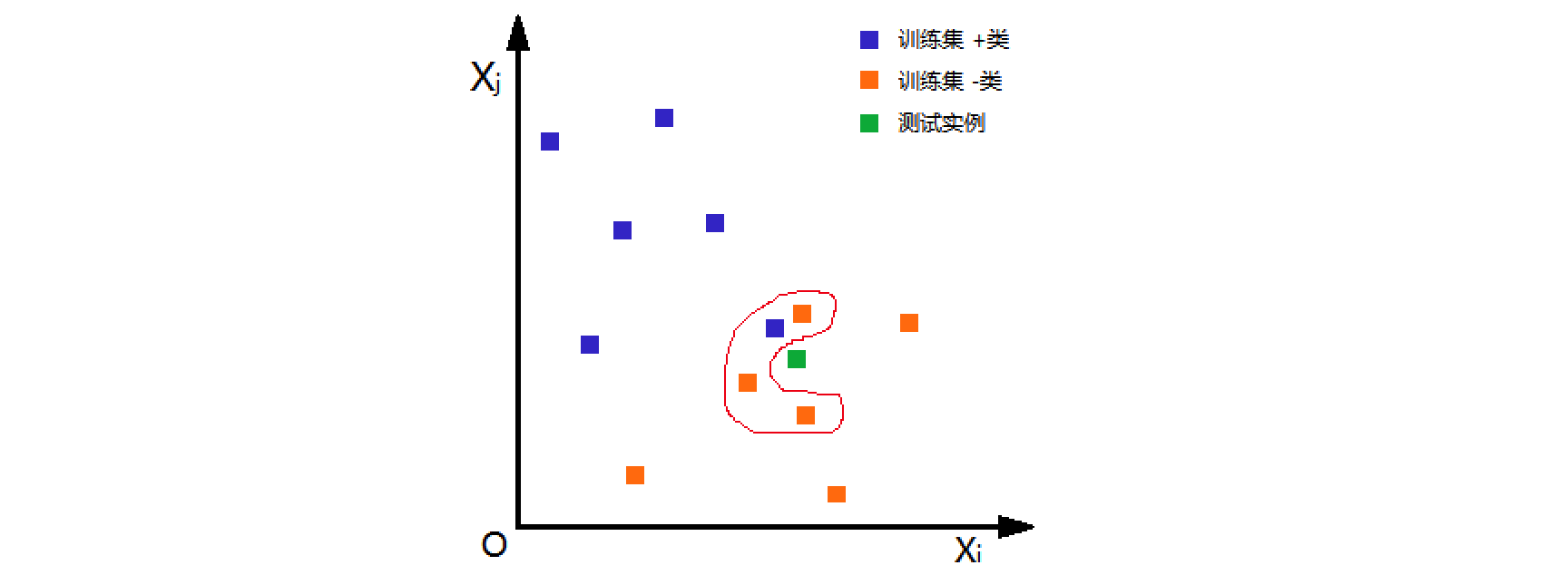图1
1.距离度量
特征空间中的两个实例点的距离是两个实例点相似程度的反映。K近邻法的特征空间一般是n维实数向量空间Rn。使用的距离是欧氏距离，但也可以是其他距离，如更一般的Lp距离或Minkowski距离。
设特征空间X是n维实数向量空间 RnR^n，xi,xj∈Xx_i,x_j∈X，xi=(xi(1),xi(2),⋯&ThinSpace;,xi(n))Tx_i=(x_i^{(1)},x_i^{(2)},\cdots,x_i^{(n)})^T，xj=(xj(1),xj(2),⋯&ThinSpace;,xj(n))Tx_j=(x_j^{(1)},x_j^{(2)},\cdots,x_j^{(n)})^T，xi,xjx_i,x_j的LpL_p 距离定义为Lp(xi,xj)=(∑l=1n∣xi(l)−xj(l)∣p)1pL_p(x_i,x_j)=(\sum_{l=1}^{n}|{x_i}^{(l)}-{x_j}^{(l)}|^p)^{\frac{1}{p}}这里p≥1。
当p=1时，称为曼哈顿距离（Manhattan distance），即L1(xi,xj)=∑l=1n∣xi(l)−xj(l)∣L_1(x_i,x_j)=\sum_{l=1}^{n}|{x_i}^{(l)}-{x_j}^{(l)}|当p=2时，称为欧氏距离（Euclidean distance），即L2(xi,xj)=(∑l=1n∣xi(l)−xj(l)∣2)12L_2(x_i,x_j)=(\sum_{l=1}^{n}|{x_i}^{(l)}-{x_j}^{(l)}|^2)^{\frac{1}{2}}当p=∞时，它是各个坐标距离的最大值，即L∞(xi,xj)=maxl ∣xi(l)−xj(l)∣L_\infty(x_i,x_j)=max_l\ |{x_i}^{(l)}-{x_j}^{(l)}|证明：
以二维实数向量空间（n=2）为例说明曼哈顿距离和欧氏距离的物理意义。
① 曼哈顿距离L1(xi,xj)=∑l=12∣xi(l)−xj(l)∣=∣xi(1)−xj(1)∣+∣xi(2)−xj(2)∣L_1(x_i,x_j)=\sum_{l=1}^{2}|{x_i}^{(l)}-{x_j}^{(l)}|=|{x_i}^{(1)}-{x_j}^{(1)}|+|{x_i}^{(2)}-{x_j}^{(2)}|图2绿色线即曼哈顿距离物理意义，其中横向线条表示|x1i-x1j|，竖向线条表示|x2i-x2j|。
② 欧氏距离L2(xi,xj)=(∑l=12∣xi(l)−xj(l)∣2)12=∣xi(1)−xj(1)∣2+∣xi(2)−xj(2)∣22L_2(x_i,x_j)=(\sum_{l=1}^{2}|{x_i}^{(l)}-{x_j}^{(l)}|^2)^{\frac{1}{2}}=\sqrt{|{x_i}^{(1)}-{x_j}^{(1)}|^2+|{x_i}^{(2)}-{x_j}^{(2)}|^2}图2红色线即欧氏距离物理意义，根据勾股定理可得。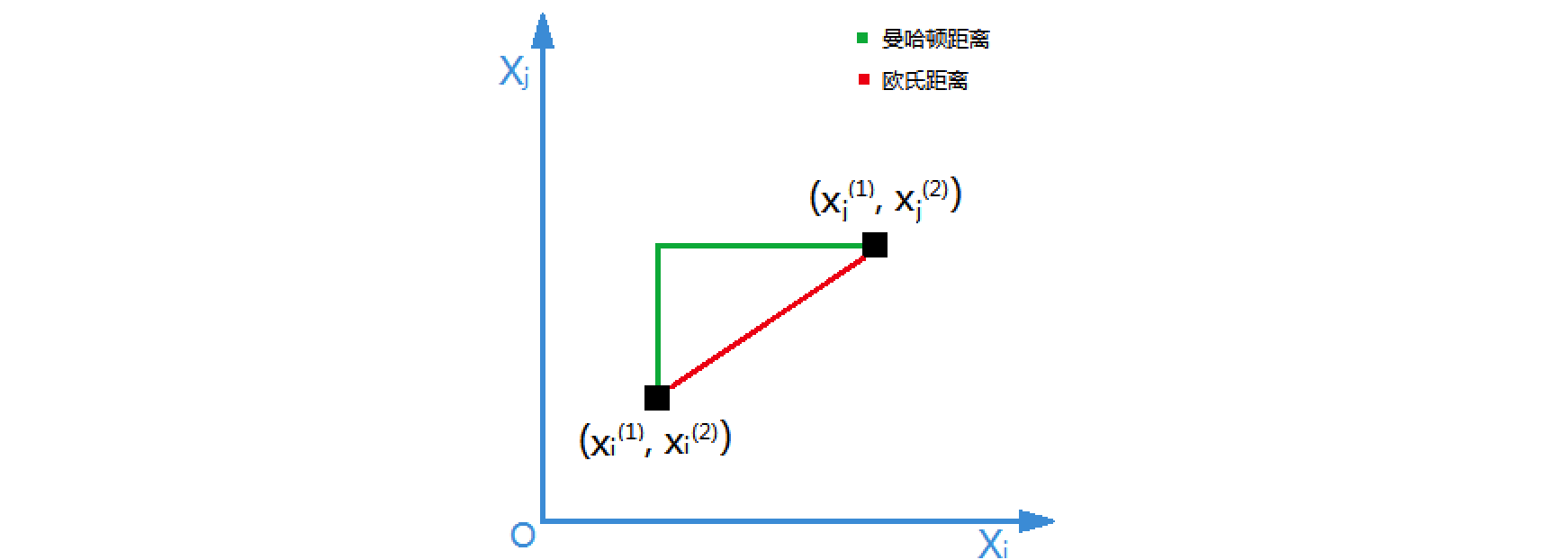图2
2.k值的选择
k值的选择会对k近邻法的结果产生重大影响。在应用中，k值一般取一个比较小的数值，通常采用交叉验证法来选取最优的k值。
3.分类决策规则
k近邻法中的分类决策规则往往是多数表决，即由输入实例的k个邻近的训练实例中的多数类，决定输入实例的类。
二、k近邻算法及代码实现（python）
1.算法
算法1（k近邻法）
输入：训练集D={(x1,y1),(x2,y2),⋯&ThinSpace;,(xN,yN)}D=\{(x_1,y_1),(x_2,y_2),\cdots,(x_N,y_N)\}
其中，xi∈X⊆Rnx_i∈X ⊆ R^n为实例的特征向量，yi∈Y={c1,c2,⋯&ThinSpace;,ck}y_i∈Y=\{c_1,c_2,\cdots,c_k\}为实例的类别，i=1,2,…,N。
输出：实例x所属的类别y
① 根据给定的距离度量，在训练集D中找出与x最近邻的k个点，涵盖这k个点的x的领域记作Nk(x)N_k(x)
② 在Nk(x)N_k(x)中根据分类决策规则（如多数表决）决定x的类别yy=arg maxcj∑xi∈Nk(x)I(yj=cj),     i=1,2,⋯&ThinSpace;,N;j=1,2,⋯&ThinSpace;,Ky=arg\ max_{c_j}\sum_{x_i\in N_k(x)}I(y_j=c_j),\ \ \ \ \ i=1,2,\cdots,N;j=1,2,\cdots,K
上式中，I为指示函数，即当yi=ci时I为1，否则I为0。
2.代码实现（python）
以下代码来自Peter Harrington《Machine Learing in Action》
距离度量为欧氏距离，分类决策规则为多数表决。classify0()函数有4个输入参数：用于分类的输入向量是inX，输入的训练集为dataSet，类别为labels，k表示用于选择最近邻的数目。
代码如下（保存为kNN.py）：
# -- coding: utf-8 --
form numpy import *
import operator

def createDataSet():
# 创建训练集
group = array([[1.0,1.1],[1.0,1.0],[0,0],[0,0.1]])
labels = ['A','A','B','B']
return group, labels

def classify0(inX, dataSet, labels, k):
dataSetSize = dataSet.shape
# 根据欧式距离计算训练集中每个样本到测试点的距离
diffMat = tile(inX, (dataSetSize,1)) - dataSet
sqDiffMat = diffMat**2
sqDistances = sqDiffMat.sum(axis=1)
distances = sqDistances**0.5
# 计算完所有点的距离后，对数据按照从小到大的次序排序
sortedDistIndicies = distances.argsort()
# 确定前k个距离最小的元素所在的主要分类，最后返回发生频率最高的元素类别
classCount={}
for i in range(k):
voteIlabel = labels[sortedDistIndicies[i]]
classCount[voteIlabel] = classCount.get(voteIlabel,0) + 1
sortedClassCount = sorted(classCount.iteritems(), key=operator.itemgetter(1), reverse=True)
return sortedClassCount

运行命令如下：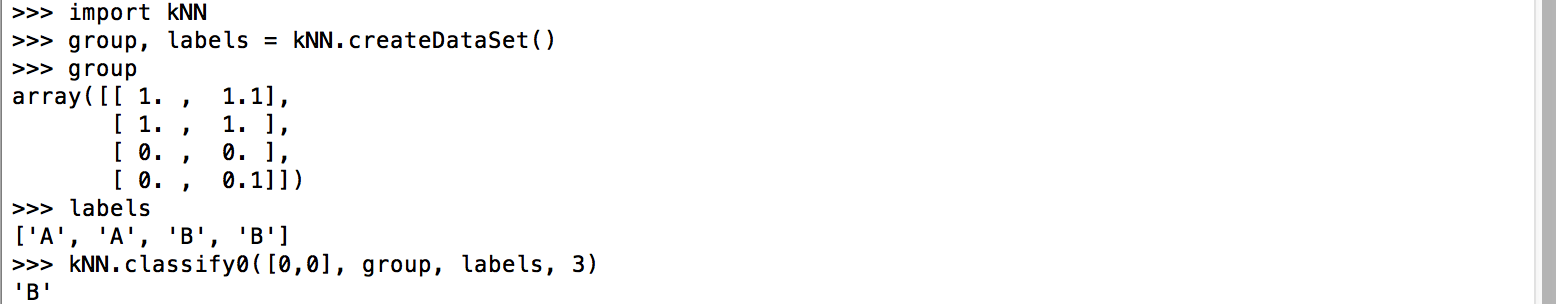group和labels为训练集，其中group为特征向量，labels为类别。输入测试实例[0,0]，选取与测试实例距离最近的3个元素，最后返回这3个元素中发生频率最高的元素类别，即为kNN近邻法的预测值。
三、k近邻法的实现：kd树
实现k近邻法时，主要考虑的问题是如何对训练数据进行快速k近邻搜索，这点在特征空间的维数大及训练数据容量大时尤其必要。
k近邻法最简单的实现方法是线性扫描（linear scan），这时要计算输入实例与每一个训练实例的距离，当训练集很大时，计算非常耗时。为了提高k近邻法搜索的效率，可以考虑使用特殊的结构存储训练数据，以减少计算距离的次数。具体方法很多，下面介绍其中的kd树方法（kd树是存储k维空间数据的树结构，这里的k与k近邻法的k意义不同）。
1.构造kd树
kd树是二叉树，是一种对k维空间中实例点进行存储以便对其进行快速检索的树形数据结构。kd树表示对k维空间的一个划分（partition），构造kd树相当于不断地用垂直于坐标轴的超平面将k维空间切分，构成一系列的k维超矩形区域。kd树的每个结点对应于一个k维超矩形区域。
通常，依次选择坐标轴对空间切分，选择训练实例点在选定坐标轴上的中位数（一组数据按大小顺序排列起来，处于中间的一个数或最中间两个数的平均值。本文在最中间有两个数时选择最大值作中位数）为切分点，这样得到的kd树是平衡的。注意，平衡的kd树搜索时未必是最优的。
2.算法
算法2（构造平衡kd树）
输入：k维空间数据集D={x1,x2,⋯&ThinSpace;,xn}D=\{x_1,x_2,\cdots,x_n\}其中xi=(xi(1),xi(2),⋯&ThinSpace;,xi(n))T，i=1,2,⋯&ThinSpace;,Nx_i=(x_i^{(1)},x_i^{(2)},\cdots,x_i^{(n)})^T，i=1,2,\cdots,N
输出：kd树
① 开始：构造根结点，根结点对应于包含D的k维空间的超矩形区域。
选择x1为坐标轴，以D中所有实例的x1坐标的中位数为切分点，将根结点对应的超矩形区域切分为两个子区域。切分由通过切分点并与坐标轴x1垂直的超平面实现。
由根结点生成深度为1的左、右子结点：左子结点对应坐标x1小于切分点的子区域，右子结点对应坐标x1大于切分点的子区域。将落在切分超平面上的实例点保存在根结点。
② 重复：对深度为j的结点，选择xl为切分的坐标轴，l=j(mod k)+1，以该结点的区域中所有实例点的xl坐标的中位数为切分点，将该结点对应的超矩形区域切分为两个子区域。切分由通过切分点并与坐标轴xl垂直的超平面实现。
由该结点生成深度为j+1的左、右子结点：左子结点对应坐标xl小于切分点的子区域，右子结点对应坐标xl大于切分点的子区域。将落在切分超平面上的实例点保存在该结点。
③ 直到两个子区域没有实例存在时停止，从而形成kd树的区域划分。例1 给定一个二维空间的数据集：D={(2,3)T,(5,4)T,(9,6)T,(4,7)T,(8,1)T,(7,2)T}
D=\{(2,3)^T,(5,4)^T,(9,6)^T,(4,7)^T,(8,1)^T,(7,2)^T\}
构造一个平衡kd树。
解：D为二维空间，则k=2。
① D中6个实例的x1坐标的中位数为7，则以(7 , 2)为切分点，由通过切分点并与坐标轴x1垂直的平面将超矩形区域切分为两个子区域。根结点为(7 , 2)，左区域包括：(2 , 3) , (5 , 4) ,  (4 , 7)，右区域包括：(8 , 1) , (9 , 6)，深度为1
② 对深度为j=1的结点，选择l=j(mod k)+1=1(mod 2)+1=2即x2为切分的坐标轴。则左区域的切分点为(5 , 4) ，左子区域为(2 , 3)，右子区域为(4 , 7)；右区域的切分点为(9 , 6) ，左子区域为(8 , 1)。
如此递归，最后得到的平衡kd树如下所示：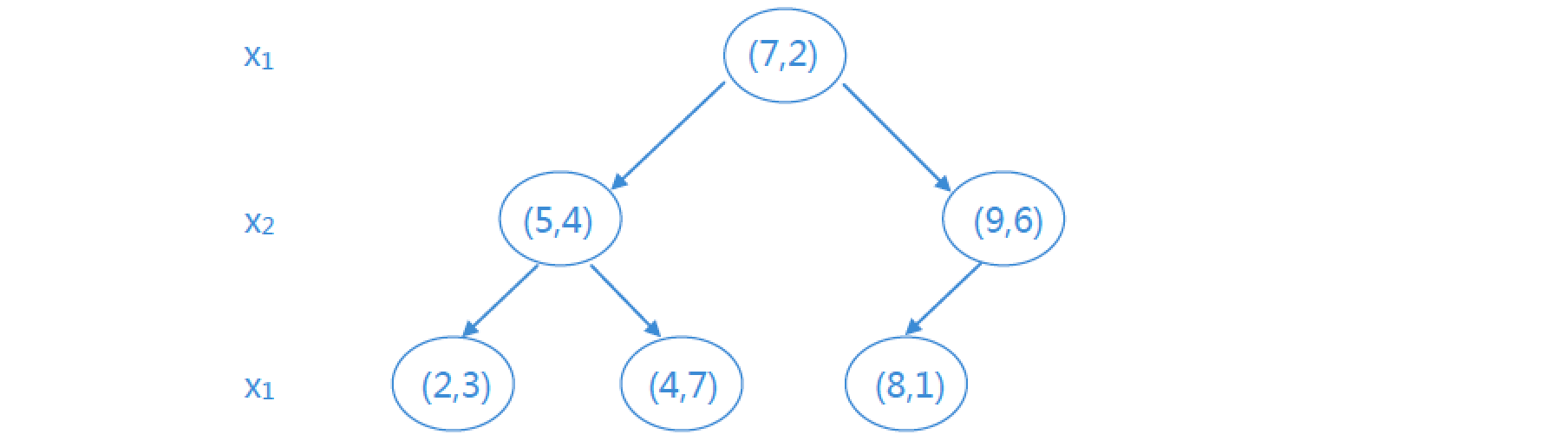3.搜索kd树（未完，待续）
以上全部内容参考书籍如下：
李航《统计学习方法》
周志华《机器学习》
Peter Harrington《Machine Learing in Action》


展开全文机器学习
• ## 机器学习之KNN（k近邻）算法详解

万次阅读 多人点赞 2020-07-19 08:13:57
1-1 机器学习算法分类 一、基本分类： ①监督学习（Supervised learning） 数据集中的每个样本有相应的“正确答案”， 根据这些样本做出 预测， 分有两类： 回归问题和分类问题。 步骤1： 数据集的创建和...
• 机器学习 从广义上讲，机器学习是一种能够赋予机器学习的能力，让它以此完成直接编程无法完成的功能的方法 从实践的意义上讲，机器学习是一种通过利用数据，训练出模型，然后使用模型预测的一种方法 人类的学习是一...python sklearn
• ## 机器学习之KNN最邻近分类算法

万次阅读 多人点赞 2019-04-05 18:42:39
KNN算法简介 KNN（K-Nearest Neighbor）最邻近分类算法是数据挖掘分类（classification）技术中最简单的算法之一，其指导思想是”近朱者赤，近墨者黑“，即由你的邻居来推断出你的类别。 KNN最邻近分类算法的...最邻近算法 python
• KNN机器学习入门原理机器学习分类1. 有监督学习（知道结果）分类 （有限数据）回归 （无限数据）2. 无监督学习聚类3. 半监督学习（不用管）深度学习KNN 基础知识k-近邻算法原理适用数据范围：优缺点&改进KNN ...
• 机器学习实战（一） KNN 本人，研一机器学习和数据挖掘的课比较少，加上对机器学习比较感兴趣，本科也接触了一些知识和项目，发现很多算法都是直接调用库，实现很少实操，特此把每个算法的步骤都复现一遍，算是加强...机器学习 数据挖掘 算法
• 机器学习笔记-KNN 0x00 系列文章目录 机器学习笔记-KNN 机器学习笔记-决策树 0x01 摘要 K近邻(KNN)，全名为k nearest neighbours，最近的K个邻居。 核心思想是找到目标节点最近的K个样本点，将他们的Y值...机器学习
• KNN（最邻近规则分类K-Nearest-Neighibor)KNN算法 1. 综述 1.1 Cover和Hart在1968年提出了最初的邻近算法 1.2 分类(classification)算法 1.3 输入基于实例的学习(instance-based learning), 懒惰学习(lazy ...
• 1.过程:计算测试样本与训练样本之间的距离，这里的距离有欧式距离，曼哈顿距离，拉普拉斯距离等。按照距离进行排序选择其中最近的k个值，这里k值的选择用到交叉验证的方法，交叉验证包括s折，随机，留一根据分类决策...
• 本文直接给出sklearn里面KNN 算法的用法。具体实现过程如下： import numpy as np from sklearn import datasets import operator from sklearn import neighbors import sklearn.model_selection as ms ...
• KNN算法思路简洁，但是在实践中却相当有效。它不仅可以用于分类，还可以用于回归，但主要应用于分类。在此前的文章中，我们给出的实例是基于Matlab实现的。本文将演示在Python语言中利用scikit-learn提供的函数来...KNN回归 Python 机器学习
• KNN算法概述     k近邻（简称KNN）算法是一种基本的分类与回归方法。工作机制：给定测试样本，基于某种距离度量找出训练集中与其最靠近的K个训练样本，然后基于这K个”邻居“的信息来预测。 &...机器学习
• 理论部分与“机器学习算法与python实践：k近邻(kNN)”这篇博文相同，实践数据也相同，差别为代码部分为作者用Matlab重新编写。 最近开始学习机器学习，理论部分主要参考周志华老师的《机器学习》这本书，实践部分...
• knn（k近邻模型）： 一种简单的分类模型 分类依据： 看离待分点最近的K个邻居属于哪个分类的最多，通过调整K的值，可能会得到不同的分类效果 步骤： 1.给出已标注好的数据点i(i=1,…,n)的坐标（xi,yix_i,y_ixi​,yi...
• 超参数：运行机器学习算法前需要指定的参数； kNN算法中的超参数：k、weights、P； 一般超参数之间也相互影响； 调参，就是调超参数； 　1）问题 　# 以kNN算法为例 平票：如果k个点中，不同类型...
• 刚刚开始在一个视频上学习机器学习，不懂的还是很多，这也算作是学习机器学习的笔记吧 KNN算法，K nearest neighbor 最近的K个邻居，了解一个算法，先从了解一个问题开始，现在问题如下，有很多的数字图片，每个...
• 生成数据：首先建立一个模块KNN.py，写一个生成数据的函数from numpy import * import operator def createDataSet(): group = array([[1.0,1.1],[1.0,1.0],[0,0],[0,0.1]]) labels = ['A','A','B','B'] return ...机器学习 机器学习实战
...